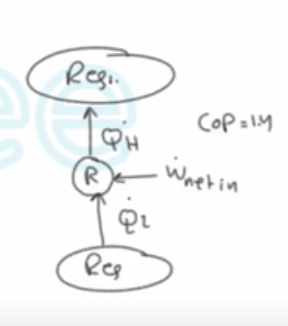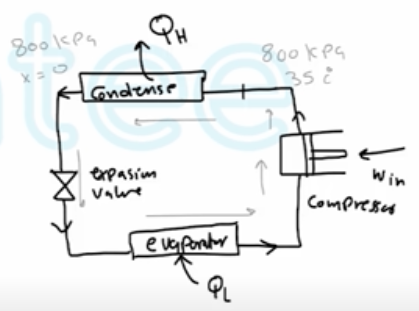Need Help?

Subscribe to Thermodynamics

###### \${selected_topic_name}
• Notes
• Comments & Questions

$\begin{array}{l}{\text { A refrigerator used to cool a computer requires } 3 \mathrm{kW} \text { of electrical power and has a COP of } 1.4 .} \\ {\text { Calculate the cooling effect of this refrigerator, in } \mathrm{kW} \text { . }}\end{array}$$Cop_{(R)}=\frac{\dot{Q}_{L}}{\dot w_{net_{in}}}$

$\rightarrow \dot Q_{L}=cop * \dot{w_{net_{in}}}$

$=1.4 * 3={4.2 \mathrm{kw}}$

$\begin{array}{l}{\text { A heat pump is used to maintain a house at a constant temperature of } 23^{\circ} \mathrm{C} \text { . }} \\ {\text { The house is losing heat to the outside air through the walls and the windows }} \\ {\text { at a rate of } 60,000 \mathrm{kJ} / \mathrm{h} \text { while the energy generated within the house from people, }} \\ {\text { lights, and appliances amounts to } 4000 \mathrm{kJ} / \mathrm{h} \text { . For a } \mathrm{COP} \text { of } 2.5, \text { determine }} \\ {\text { the required power input to the heat pump. }}\end{array}$$\left.\begin{array}{l}{P_{1}=800 \mathrm{KPa}} \\ {T_{1}=35 c^{\circ}}\end{array}\right]\rightarrow h_1=271.42 kJ/kg$

$\left.\begin{array}{l}{P_{2}=800 \mathrm{kPa}} \\ {X_{2}=0}\end{array}\right] \rightarrow h_{2}=95.48 \mathrm{kJ} / \mathrm{kg}$

$\dot{Q}_{H}=\dot{m}\left(h_{1}-h_{2}\right)$

$=(0.018)(271.24-95.48)$

$=3.164 \mathrm{kw}$

$Cop_{(H P)}=\frac{\dot{Q}_ H}{\dot W_{i n}}=\frac{3.164}{1.2}=2.64$

$\dot{Q}_{L}=\dot{Q}_{H}-\dot{W}_{i n}=3.164-1.2=1.96 \mathrm{kw}$

No comments yet